# A SAMPLE L TEX DOCUMENT

## Transcription

A SAMPLE L TEX DOCUMENT
A SAMPLE LATEX DOCUMENT
DAVID HANDRON AND JAMES CUMMINGS
Abstract. This is a document to highlight the main features of LATEX.
This can be used as a template when producing your own documents.
Documents (for example homework solutions) often contain numbered lists.
These are easy to make with LATEX.
(1) Yes.
(2) No.
(3) Maybe.
You can override the default numbering scheme.
eins
zwei
drei
vier
Yes.
No.
Maybe.
Maybe not.
Numbered lists can contain sublists.
(1) (a) Yes.
(b) No.
(2) Maybe.
1. Setting mathematical formulae in LATEX
2
Consider the equation y = xa+n1 .
Compare with the equation y = xa + n21 .
Here is a displayed formula.
ex = 1 + x +
x2 x3
+
+ ...
2
3
Here is a formula containing ordinary text.
E = {n : n is even}
Date: August 28, 2004.
1
2
DAVID HANDRON AND JAMES CUMMINGS
2. Examples: typesetting calculus and linear algebra
2.1. Limits. Here is the definition of a limit.
Definition 1. We say that limx→a = L if given any , there is a δ such that
|f (x) − L| < whenever |x − a| < δ.
Note the difference between limx→a = L and
lim = L.
x→a
LATEX knows to save space in the inline equation by writing the limit x → a
as a subscript. In the displayed equation, x → a is placed under the limit,
where it belongs.
2.2. Derivatives. There is no specific symbol in LATEX for a derivative, but
it can be built up by making a fraction:
dy
.
dx
So we might typeset something like
y(x) =
dy
dx =
√
x2 + 1
√ x
x2 +1
Notice how I have used an array to line up the equations at the equal signs.
There are other more sophisticated ways to make things line up, but usually
an array can be made to suffice.
Sometimes we want to use different notation to denote a derivative. Given a
function f (x), we denote the derivative by “f -prime”. Hence we have f 0 (x).
The second derivative is f 00 (x). To talk about the seventh derivative we
would use the symbol f (7) (x).
Rb
2.3. Integration. Here is a nice integration problem: a (x2 − x)dx. And
here is the solution:
b
Rb 2
x2 x3
x
−
xdx
=
−
a
a
3 3 2 2 3
b
b
a
a2
−
=
−
−
3
2
3
2
=
2b3 −3b2
6
−
2a3 −3a2
.
6
Anyone who has taken vector calculus has seen double and triple integrals
and path integrals. LATEX has symbols for these as well:
ZZ
ZZZ
I
A SAMPLE LATEX DOCUMENT
3
2.4. Spacing. An initial value problem is a differential equation combined
with an initial condition.
dy
= f (t, y); y(y0 ) = t0 .
dt
This is all well and good, but looks a bit cramped. We can use the command
\quad to insert a blank space:
dy
= f (t, y)
dt
If this is not enough, use \qquad.
dy
= f (t, y)
dt
;
;
y(y0 ) = t0 .
y(y0 ) = t0 .
Another example of a spacing problem:
∀x∃yφ(x, y)
We can try with quads
∀x ∃y φ(x, y)
but that looks a bit too spaced out. The smaller space \, does the trick:
∀x ∃y φ(x, y)
2.5. Sequences and Series. The main difficulty with sequences and series
is just knowing the commands that will produce the appropriate symbols.
We can define a sequence
n ∞
2
4 8
an = 3
= 3, 2, , , . . .
3
3 9
n=0
We can add all the terms of this sequence together to get an infinite series:
n
∞
X
3
2
=
a0 + a1 + a2 + . . . + an + . . . =
3
2 =9
3
1
−
3
n=1
2.6. Matrix Algebra. Matrices are typeset by using an array command.
For instance, the rule for multiplying two 2 × 2 matrices together is given
by
a b
e f
ae + bg af + bh
=
c d
g h
ce + dg cf + dh
Now is also a good time to point out the difference between
a b
a b
and [
].
c d
c d
also between
3
4
and
3
( )
4
4
DAVID HANDRON AND JAMES CUMMINGS
3. More examples
LATEX defines many symbols which are useful in typing mathematics. The
following list illustrates the use of some of these symbols along with a few
other important points. A nice list of symbols can be found on the web at
http://students.uniyar.ac.ru/~tex/lshort/node52.html
• a0 ≤ a1 ≤ a2 ≤ . . .
• Let F : X → Y be a function and let X0 ⊆ X. Then F X0 is the
function which has domain X0 and agrees with F on that domain.
• Given any two sets X and Y the Cartesian product X × Y is the set
of ordered pairs (x, y) with x ∈ X and y ∈ Y .
• If X and Y are sets then X * Y if and only if X \ Y 6= ∅.
• Consider a strictly increasing sequence of sets X0 ( X1 ( X2 . . ..
Here is a definition by cases.

2

x − c, if x ≤ 3
f (x) = x2 + c, if 3 < x ≤ 5

 2
x − c, otherwise
Here is an example of a theorem with a proof (the proof environment is built
into the AMS article class so we did not need to create it ourselves).
Theorem 1. A countable union of countable sets is countable.
Proof. Let fn : N Xn for all n ∈ N. Define a function f : N →
f : 2m (2n + 1) − 1 7→ fm (n) and check this works.
S
n Xn
by


### Latex Gauntlet Pattern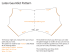More information

### 15-322 Retail Light-Fit - UIS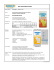More information

### GEL-TEK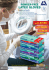More information

### The esrelation Package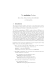More information

### Document 6509150More information

### Class 1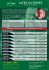More information

### Latexco Solutions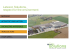More information

### RL2008 (Geltex)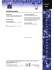More information

### neopreno TRAD AN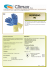More information

### A Sample ACM SIG Proceedings Paper in LaTeX Format Pat Trovato ABSTRACT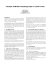More information

### Cariflexâ¢ Polyisoprene Latex: Pure Performance and Comfort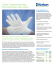More information

### HP Latex 310 Printer (1.37 m / 54 in)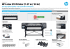More information

### HP Latex 330 Printer (1.63 m / 64 in)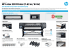More information

### SUBMISSION CHECKLIST Is your paper paper newsworthy? Learn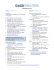More information

### - Journal of Biological and Chemical Research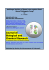More information

### When grace meets beauty â LaTeX meets mathematics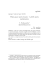More information

### AN OPERATING MANUAL The Vision Laboratory Volen Center for Complex Systems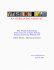More information

### Document 6597180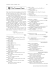More information

### Madoko Reference - Microsoft Research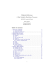More information

### chemgreek_en.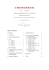More information

### User’s manual for Writer2LaTeX, Writer2BibTeX, Writer2xhtml and Calc2xhtml version 1.2.1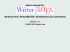More information

### International Journal of Ayurveda and Pharma Research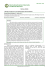More information